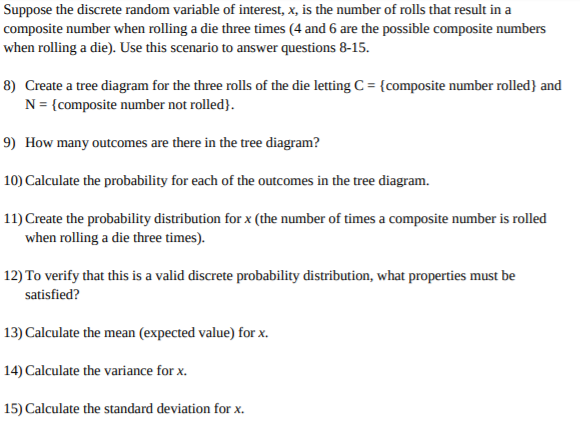# Suppose the discrete random variable of interest, x, is the number of rolls that result in acomposite number when rolling a die three times (4 and 6 are the possible composite numberswhen rolling a die). Use this scenario to answer questions 8-15.8) Create a tree diagram for the three rolls of the die letting C= {composite number rolled} andN = {composite number not rolled).9) How many outcomes are there in the tree diagram?10) Calculate the probability for each of the outcomes in the tree diagram.11) Create the probability distribution for x (the number of times a composite number is rolledwhen rolling a die three times).12) To verify that this is a valid discrete probability distribution, what properties must besatisfied?13) Calculate the mean (expected value) for x.14) Calculate the variance for x.15) Calculate the standard deviation forx

Question

need answers to questions 9 and 10help_outlineImage TranscriptioncloseSuppose the discrete random variable of interest, x, is the number of rolls that result in a composite number when rolling a die three times (4 and 6 are the possible composite numbers when rolling a die). Use this scenario to answer questions 8-15. 8) Create a tree diagram for the three rolls of the die letting C= {composite number rolled} and N = {composite number not rolled). 9) How many outcomes are there in the tree diagram? 10) Calculate the probability for each of the outcomes in the tree diagram. 11) Create the probability distribution for x (the number of times a composite number is rolled when rolling a die three times). 12) To verify that this is a valid discrete probability distribution, what properties must be satisfied? 13) Calculate the mean (expected value) for x. 14) Calculate the variance for x. 15) Calculate the standard deviation forx fullscreen
check_circleExpert Solution
Step 1

The tree diagram for the three rolls of the die letting C= {composite number rolled} and N={composite number not rolled}.

Step 2

9.

There are eight outcomes in the t...

### Want to see the full answer?

See Solution

#### Want to see this answer and more?

Solutions are written by subject experts who are available 24/7. Questions are typically answered within 1 hour*

See Solution
*Response times may vary by subject and question
Tagged in

### Statistics